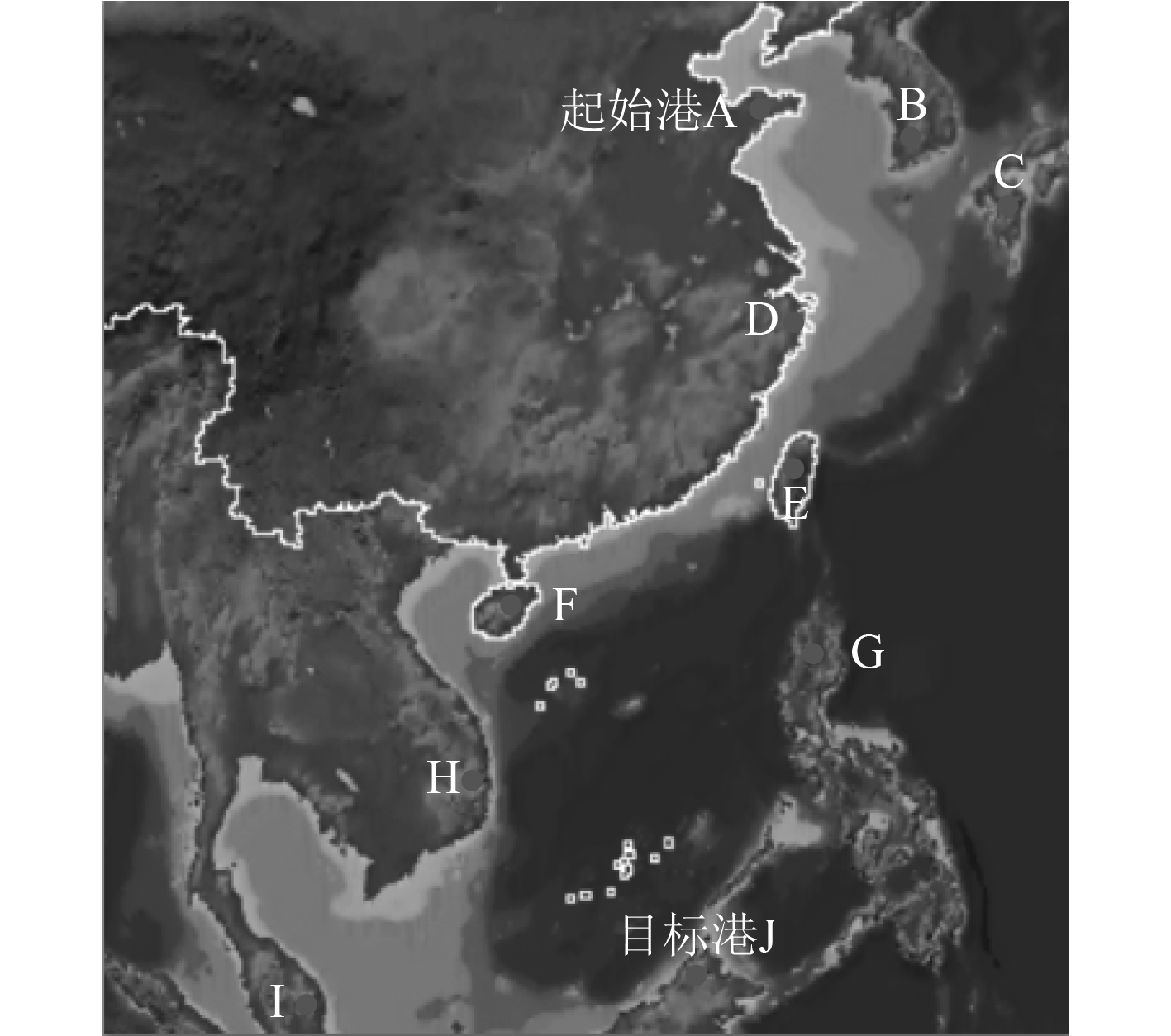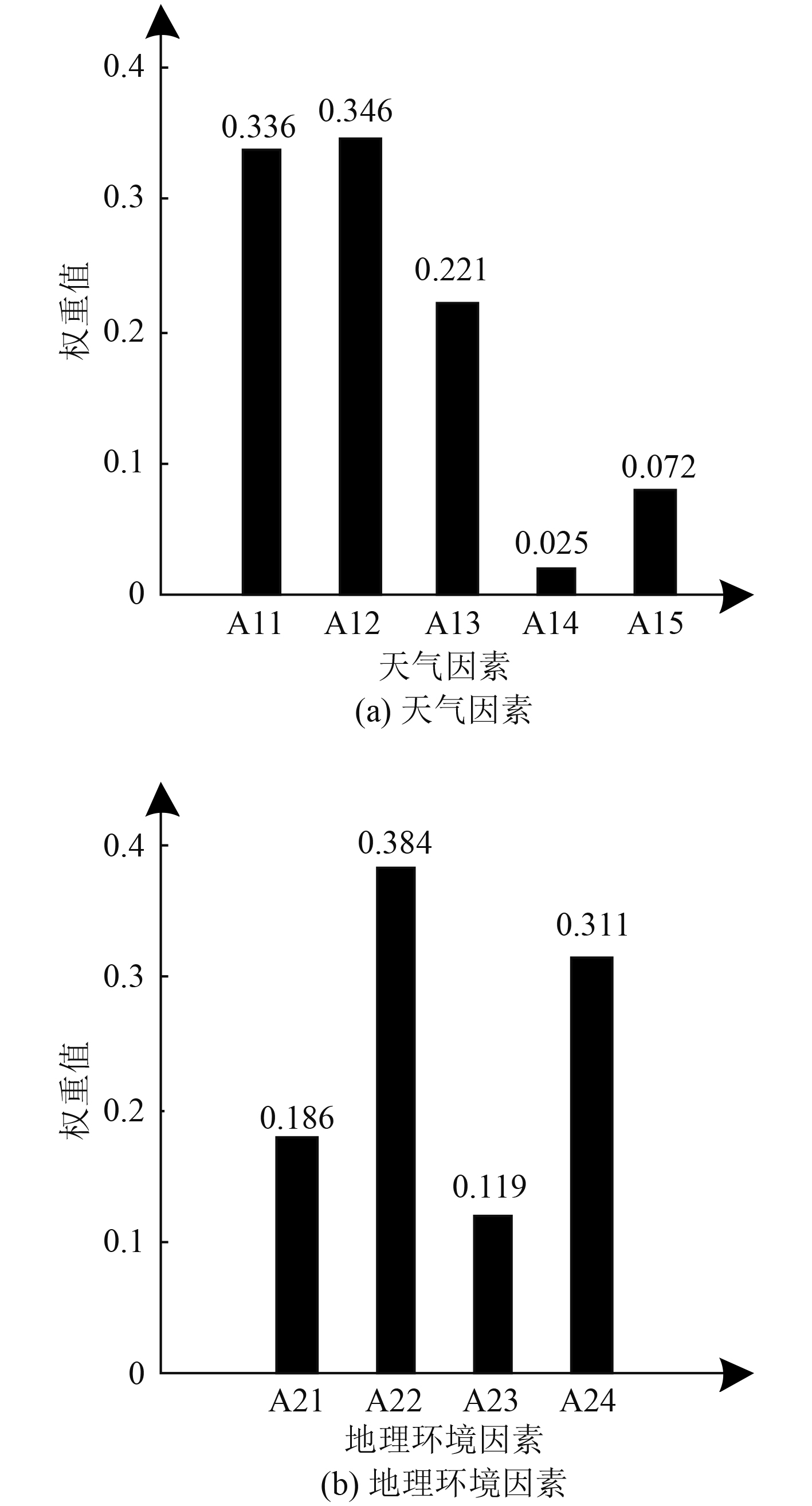﻿ 应用数据挖掘技术的舰船最佳航线自动选取方法
 舰船科学技术2022, Vol. 44Issue (9): 79-82    DOI: 10.3404/j.issn.1672-7649.2022.09.016PDF

Automatic selection method of ship optimal route based on data mining technology
JIANG Guang-kun
Dalian Ocean University, Dalian 116300, China
Abstract: In order to reduce the sailing risk, sailing time and energy consumption of ships, this study uses data mining technology to design the automatic selection method of ship's optimal route. Based on weather factors and geographical environment factors, ice density risk index and channel water depth risk index are selected, and ship route risk assessment index system is constructed. Then the fuzzy evaluation method in data mining technology is used to evaluate the navigation risk of each route and exclude the routes whose risk level is above medium. An undirected map of the traffic network is constructed to describe the routes with medium risk level and below. A* algorithm in data mining technology is used to obtain the shortest route, which is the best route for ships. The application results show that this method can accurately evaluate the route risk, and effectively reduce the sailing risk, sailing time and energy consumption of ships.
Key words: data mining     optimal ship route     automatic selection     risk assessment     index system     shortest path
0 引　言

1 舰船最佳航线自动选取方法

1.1 舰船航线风险评估 1.1.1 风险评估指标体系构建表 1 舰船航线风险评估指标体系构建 Tab.1 Construction of ship route risk assessment index system

1）A11：其值可通过航线区域的天气预报数据获取，用于描述冰的密集度对于舰船航行的威胁程度，同舰船航线风险之间呈正比例相关。

2）A12：用于描述舰船航线内冰密集度低于40%的天数，其值同舰船航线风险之间呈反比例相关。A12的量化描述为：

 $A12{\text{ = }}\frac{B}{{{B_{all}}}} \times \phi。$ (1)

3）A13：用于描述低能见度对舰船航线的威胁程度，其值同舰船航线风险之间呈正比例相关。A13量化描述为：

 $A13{\text{ = }}\left( {\frac{{{Z_0}}}{Z}} \right) \times \left( {\frac{{{X_n}}}{Y}} \right) 。$ (2)

4）A14：用于描述大风对舰船航线的威胁程度，其值同舰船航线风险之间呈正比例相关。A14的量化描述为：

 $A14{\text{ = }}\left( {\frac{W}{{{W_{\min }}}}} \right)\left( {\frac{{{X_f}}}{Y}} \right) 。$ (3)

5）A15：用于描述气温对舰船航线的威胁程度，其值同舰船航线风险之间呈反比例相关。A15的量化描述为：

 $A15=\left\{\begin{array}{*{20}{l}}0&Q > 0^{\circ}C，\\ \left|\dfrac{Q}{0-{Q}_{\mathrm{min}}}\right|&Q\leqslant 0^{\circ}C。\end{array}\right.$ (4)

6）A21：用于描述航道水深对航行舰船的威胁程度，该值同舰船航行过程中触礁与搁浅的风险之间呈反比例相关。A21的量化描述为：

 $A21 = \left\{ \begin{array}{*{20}{l}} 0& \dfrac{{Num\left( {{d_h} \geqslant E} \right)}}{{Num\left( {all} \right)}} \geqslant 0.6，\\ 1 - \dfrac{D}{E}&0 < \dfrac{{Num\left( {{d_h} \geqslant E} \right)}}{{Num\left( {all} \right)}} < 0.6 。\end{array} \right.$ (5)

7）A22：用于描述航道宽度对航行舰船的威胁程度，其值同舰船航线风险之间呈反比例相关。A22的量化描述为：

 A22 = \left\{ \begin{aligned} &0&{w_h} \geqslant H ，\\ &1 - \frac{{{w_h}}}{H} & 0 \leqslant {w_h} < H 。\end{aligned} \right. (6)

8）A23：用于描述航道周边岛屿对航行舰船的威胁程度，其值同舰船航线风险之间呈正比例相关。A23的量化描述为：

 $A23 = \frac{{{M_d}}}{M} \times \phi 。$ (7)

9）A24：用于描述暗礁对航行舰船的威胁程度，其值同舰船航线风险之间呈正比例相关。A24的量化描述为：

 $A24 = \frac{{{M_a}}}{M} \times \phi。$ (8)

1.1.2 基于模糊评估的风险评估

 ${K_i} = {W_i} \times {R_i} 。$ (9)

 $K = W \times R 。$ (10)
1.1.3 评估结果处理

 $v = \frac{{\displaystyle\sum\limits_{j = 1}^m {{k_j}{v_j}} }}{{\displaystyle\sum\limits_{j = 1}^m {{k_j}} }} 。$ (11)

1.2 基于A*算法的航程最短航线确定

 $l\left( {{o_i},{o_j}} \right) = l\left( {{o_i},{o_k}} \right) + l\left( {{o_k},{o_j}} \right) 。$ (12)

 $\min L = \sum\limits_{{o_i},{o_j} \in {p^ * }} {\left( {{o_i},{o_j}} \right)} 。$ (13)

2 应用结果 2.1 应用对象相关信息图 1 应用对象航行区域 Fig. 1 Navigation area of application object
2.2 航线风险评估图 2 舰船航线风险评估指标权重计算结果 Fig. 2 Calculation results of weight of ship route risk assessment index

2.3 实际应用测试表 2 应用对象事故发生率航行时间等对比结果 Tab.2 Comparison results of accident rate and sailing time of application object

3 结　语

  徐振烊, 张静远, 王鹏. 水下地形辅助导航中匹配航路的选取[J]. 系统工程与电子技术, 2019, 41(11): 2588-2596.  王莉莉, 蒋一森. 基于航程加权的航路网络效能仿真分析[J]. 计算机仿真, 2019, 36(6): 120-124+159. DOI:10.3969/j.issn.1006-9348.2019.06.024  韩志豪, 汪益兵, 张宇, 等. 基于深度强化学习的船舶航线自动规划[J]. 中国航海, 2021, 44(1): 100-105. DOI:10.3969/j.issn.1000-4653.2021.01.017  吕进锋, 马建伟, 李晓静. 基于改进的随机路径图及和声算法的舰船航线规划[J]. 控制理论与应用, 2020, 37(12): 2551-2559.